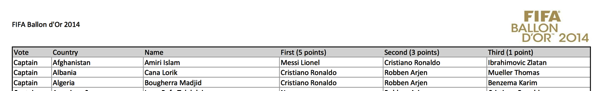# Python/pdfquery: Scraping the FIFA World Player of the Year votes PDF into shape

Last week the FIFA Ballon d'Or 2014 was announced and along with the announcement of the winner the individual votes were also made available.

Unfortunately they weren't made open in a way that Ben Wellington (of IQuantNY fame) would approve of - the choice of format for the data is a PDF file!

I wanted to extract this data to play around with it but I wanted to automate the extraction as I'd done when working with Google Trends data.

I had a quick look for PDF scraping libraries in Python and R and eventually settled on Python's pdfquery, mainly because there was lots of documentation which made it easy to get started.

One way you scrape data from a PDF is by locating an element on the page and then grabbing everything within a bounded box relative to that element.

In my case I had 17 pages all of which had a heading for each of six columns.I wanted to grab the data in each of those columns but initially struggled working out what elements I should be looking for until I came across the following function which allows you to dump an XML version of the PDF to disk:

``````
import pdfquery
pdf = pdfquery.PDFQuery("fboaward_menplayer2014_neutral.pdf")
``````

The output looks like this:

``````
<pdfxml ModDate="D:20150110224554+01'00'" CreationDate="D:20150110224539+01'00'" Producer="Microsoft&#174; Excel&#174; 2010" Creator="Microsoft&#174; Excel&#174; 2010">
<LTPage bbox="[0, 0, 841.8, 595.2]" height="595.2" pageid="1" rotate="0" width="841.8" x0="0" x1="841.8" y0="0" y1="595.2" page_index="0" page_label="">
<LTAnon> </LTAnon>
<LTTextLineHorizontal bbox="[31.08, 546.15, 122.524, 556.59]" height="10.44" width="91.444" word_margin="0.1" x0="31.08" x1="122.524" y0="546.15" y1="556.59"><LTTextBoxHorizontal bbox="[31.08, 546.15, 122.524, 556.59]" height="10.44" index="0" width="91.444" x0="31.08" x1="122.524" y0="546.15" y1="556.59">FIFA Ballon d'Or 2014 </LTTextBoxHorizontal></LTTextLineHorizontal>
<LTAnon> </LTAnon>
<LTAnon> </LTAnon>
<LTAnon> </LTAnon>
<LTAnon> </LTAnon>
<LTAnon> </LTAnon>
<LTAnon> </LTAnon>
``````

Having scanned through the file I realised that what I needed to do was locate the 'LTTextLineHorizontal' element for each heading and then grab all the 'LTTextLineHorizontal' elements that appeared in that column.

I started out by trying to grab the 'Name' column on the first page:

``````
>>> name_element = pdf.pq('LTPage[pageid=\'1\'] LTTextLineHorizontal:contains("Name")')
>>> name_element.text
'Name '
``````

Next I needed to get the other elements in that column. With a bit of trial and error I ended up with the following code:

``````
x = float(name_element.get('x0'))
y = float(name_element.get('y0'))
cells = pdf.extract( [
('with_parent','LTPage[pageid=\'1\']'),
('cells', 'LTTextLineHorizontal:in_bbox("%s,%s,%s,%s")' % (x, y-500, x+150, y))
])

>>> [cell.text.encode('utf-8').strip() for cell in cells['cells']]
['Amiri Islam', 'Cana Lorik', 'Bougherra Madjid', 'Luvu Rafe Talalelei', 'Sonejee Masand Oscar', 'Amaral Felisberto', 'Liddie Ryan', 'Griffith Quinton', 'Messi Lionel', 'Berezovskiy Roman', 'Breinburg Reinhard', 'Jedinak Mile', 'Fuchs Christian', 'Sadigov Rashad', 'Gavin Christie', 'Hasan Mohamed', 'Mamun Md Mamnul Islam', 'Burgess Romelle', 'Kalachou Tsimafei', 'Komany Vincent', 'Eiley Dalton', 'Nusum John', 'Tshering Passang', 'Raldes Ronald', 'D\xc5\xbeeko Edin', 'Da Silva Santos Junior Neymar', 'Ceasar Troy', 'Popov Ivelin', 'Kabore Charles', 'Ntibazonkiza Saidi', 'Kouch Sokumpheak']
``````

I cleaned that up and generified it to work for any page and for columns of different widths. This is what the function looks like:

``````
name_element = pdf.pq('LTPage[pageid=\'%s\'] LTTextLineHorizontal:contains("%s")' % (page, header))
x = float(name_element.get('x0'))
y = float(name_element.get('y0'))
cells = pdf.extract( [
('with_parent','LTPage[pageid=\'%s\']' %(page)),
('cells', 'LTTextLineHorizontal:in_bbox("%s,%s,%s,%s")' % (x, y-500, x+cell_width, y))
])
return [cell.text.encode('utf-8').strip() for cell in cells['cells']]
``````

We can then call that for each column on the page and zip together the resulting arrays to get a tuple for each row:

``````
roles = extract_cells(1, "Vote", 50)
countries = extract_cells(1, "Country", 150)
voters = extract_cells(1, "Name", 170)
first = extract_cells(1, "First (5 points)", 150)
second = extract_cells(1, "Second (3 points)", 150)
third = extract_cells(1, "Third (1 point)", 130)

>>> for vote in zip(roles, countries, voters, first, second, third)[:5]:
print vote

('Captain', 'Afghanistan', 'Amiri Islam', 'Messi Lionel', 'Cristiano Ronaldo', 'Ibrahimovic Zlatan')
('Captain', 'Albania', 'Cana Lorik', 'Cristiano Ronaldo', 'Robben Arjen', 'Mueller Thomas')
('Captain', 'Algeria', 'Bougherra Madjid', 'Cristiano Ronaldo', 'Robben Arjen', 'Benzema Karim')
('Captain', 'American Samoa', 'Luvu Rafe Talalelei', 'Neymar', 'Robben Arjen', 'Cristiano Ronaldo')
('Captain', 'Andorra', 'Sonejee Masand Oscar', 'Cristiano Ronaldo', 'Mueller Thomas', 'Kroos Toni')
``````

The next step was to write out each of those rows to a CSV file so we can use it from another program. The full script looks like this:

``````
import pdfquery
import csv

name_element = pdf.pq('LTPage[pageid=\'%s\'] LTTextLineHorizontal:contains("%s")' % (page, header))
x = float(name_element.get('x0'))
y = float(name_element.get('y0'))
cells = pdf.extract( [
('with_parent','LTPage[pageid=\'%s\']' %(page)),
('cells', 'LTTextLineHorizontal:in_bbox("%s,%s,%s,%s")' % (x, y-500, x+cell_width, y))
])
return [cell.text.encode('utf-8').strip() for cell in cells['cells']]

if __name__ == "__main__":
pdf = pdfquery.PDFQuery("fboaward_menplayer2014_neutral.pdf")

pages_in_pdf = len(pdf.pq('LTPage'))

writer.writerow(["Role", "Country", "Voter", "FirstPlace", "SecondPlace", "ThirdPlace"])
for page in range(1, pages_in_pdf + 1):
print page
roles = extract_cells(page, "Vote", 50)
countries = extract_cells(page, "Country", 150)
voters = extract_cells(page, "Name", 170)
first = extract_cells(page, "First (5 points)", 150)
second = extract_cells(page, "Second (3 points)", 150)
third = extract_cells(page, "Third (1 point)", 130)
votes = zip(roles, countries, voters, first, second, third)